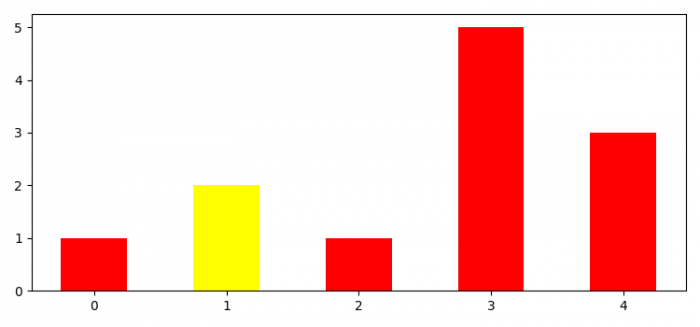# How to change the color of a single bar if a condition is true (Matplotlib)?

To change the color of a single bar if a condition is true, we can make a set of values and a list of colors with red until the value is 2; else add yellow color in the list.

## Steps

• Set the figure size and adjust the padding between and around the subplots.
• Initialize a variable width of a bar.
• Make two lists of values and colors.
• Use bar() method to plot bars.
• To display the figure, use show() method.

## Example

import numpy as np
import matplotlib.pyplot as plt
plt.rcParams["figure.figsize"] = [7.50, 3.50]
plt.rcParams["figure.autolayout"] = True
data = np.arange(5)
width = 0.5
vals = [1, 2, 1, 5, 3]
colors = ["red" if i != 2 else "yellow" for i in vals]
plt.bar(data, vals, width, color=colors)
plt.show()

## Output